# Using MADLS for Improved Component Resolution

The introduction of multi angle dynamic light scattering (MADLS) in the novel Zetasizer Ultra system offers three key benefits:

• Greater sensitivity of components in mixtures
• Greater resolution of near-in-size components (from 3:1 to 2:1)
• Conversion of the transformed number weighted size distribution into particle concentration

As these developments denote major improvements over the restrictions inherent in existing single angle DLS, it is worth offering further detail on the justifications for each.

## Dynamic Light Scattering

When a dispersion of particles is lit up by a coherent source of light, a minor portion of the light will be scattered by the particles lit up, with the force of the scattered intensity being a function of the dimensions, refractive index, and concentration of the particles, in addition to the incident light intensity and the observation angle.

The impact that these parameters bear on the scattering profile is clearly explained using Mie’s theory to Maxwell’s electromagnetic equations for homogeneous spherical scatterers, which make up the central basis of light scattering methods, including laser diffraction (LD) and static light scattering (SLS).

Figure 1 offers an example, showing the normalized scattering per spherical particle as a function of particle size and observation angle under an illuminating wavelength of 633 nm, with the vertical dashed lines signifying the 1st intensity maximum in the scattering profiles for each angle.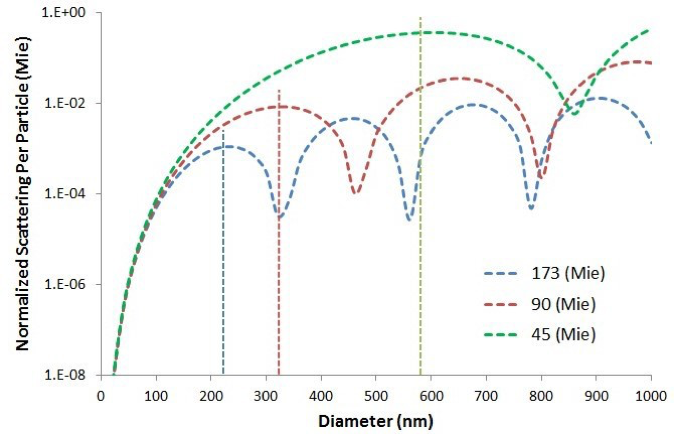Figure 1. Comparison of the size dependent scattering from a single spherical particle at 15, 90, and 173 degree scattering angles, as predicted by the Mie theory

The Mie theory relates to the ‘magnitude’ of scattered light, while Brownian motion explains the movement of particles in solution.

As such, the magnitude of light scattered from a dispersion of particles will oscillate over time, as a result of constructive and destructive wave interference, as the relative particle locations within the dispersion vary. Temporal examination of these fluctuations in intensity is the fundamental base of a method known as dynamic light scattering (DLS).

In DLS, variations in the scattering intensity are studied in relation to statistics via a method known as function analysis, with the measured correlogram signifying the time correlation of scattering intensity.

The dispersion properties which have an effect on this correlation include: the viscosity, which is inversely proportional to Brownian motion velocity; the temperature, which is linearly proportional to Brownian motion velocity; and most significantly, the size of particles, with larger particles diffusing more slowly than smaller ones and producing a measured intensity correlogram with a slower correlation decay.

Figure 2 offers an example of this, displaying the measured intensity correlation functions for dispersions of 6 nm ovalbumin and 95 nm silicon dioxide. The DLS measured correlogram comprises all the data in relation to the movement of particles lit up in the dispersion.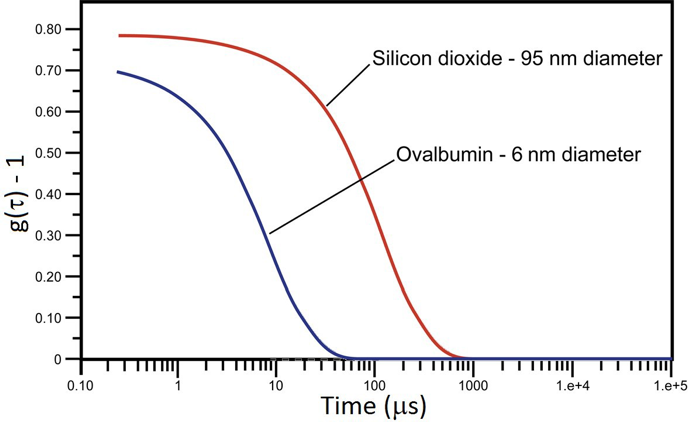Figure 2. Dynamic light scattering measured correlograms of 6 nm ovalbumin and 95 nm silicon dioxide in phosphate buffered saline. The x-axis is the delay time of the correlation function.

The intensity weighted particle size distribution of particles measured is produced through deconvolution of the correlogram, using a non-negative least squares (NNLS) fitting algorithm.

The deconvolution of the DLS measured correlogram into a particle size distribution is carried out with a NNLS fitting method in accordance with the vector expression shown below, in which g is the autocorrelation function measured at each delay time (τ), x(d) is the particle size distribution, which is intrinsically weighted by the intensity of the light scattered by each size component of the sample, and K(τ, d) is the τ and d dependent scattering matrix with τ being the autocorrelation delay time and d the particle diameter.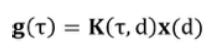## Single-Angle DLS Limitations

Despite its broad working size range from less than 1 nm to 10 μm, DLS is thought of as a low-resolution sizing method. The rule of thumb resolution limit for single angle DLS is 3 to 1 on the particle size, which means that to be able to resolve near-in-size components with DLS, they must diverge in size by a factor of 3.

The essential cause for this constraint is the inability of DLS to differentiate random noise in the correlogram from genuine signal linked to weakly scattering particles, for example, low concentration protein oligomers or particles in the region of a scattering intensity minimum, as seen in Figure 1.

Conventional NNLS algorithms attempt to make up for this by distorting the peaks in the particle size distribution, leading to the depiction as a low-resolution method.

The task of differentiating correlogram noise from genuine particle signal is especially challenging for ensuing transforms of the DLS intensity distribution to a mass or even a number distribution. Since the scattering intensity scales with the 6th power of the size, DLS intensity distributions are frequently skewed, with smaller quantities of bigger particles dictating the distribution.

As a mass distribution is frequently the hoped for outcome for scientists using DLS, the Mie theory, alongside the optical properties of the particles, is regularly employed to convert the intensity distribution into a mass or number distribution.

The central issue with these transforms is the supposition that each size bin in the intensity size distribution denotes a genuine particle family, with the band widening stemming from the inability to differentiate correlogram noise from genuine particle signals resulting in major error in the transformed mass and number distributions.

Figure 3 offers an example, displaying the intensity and volume distributions measured for a 60 nm diameter latex size standard and an oligomeric protein (BSA) mixture.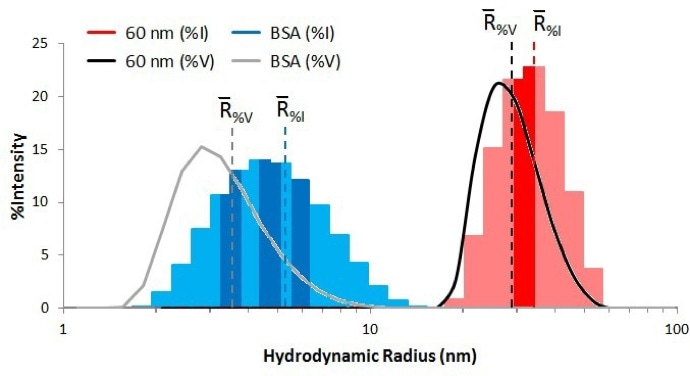Figure 3. Dynamic light scattering measured intensity (histogram) and volume (line) distributions for a 60 nm size standard and a protein (BSA), using the Zetasizer General Purpose NNLS algorithm.

In Figure 3, the intensity distributions are displayed in a histogram layout, with the bins signifying the genuine sizes of the peak components presented in the darker blue and red for BSA and latex, respectively.

The volume distributions are signified by the gray (BSA) and black (latex) curves, and the color-coded dashed lines signify the mean values for the intensity and volume weighted distributions.

It is worth noting that the latex peak has only one size component at around 30 nm radius, while the BSA peak is polydisperse, with monomers, dimers, and trimers at circa 3.5, 4.7, and 5.7 nm radii respectively, verified by size exclusion chromatography.

In the Mie transform, the intensity distribution is deemed free of errors, with each bin in the distribution histogram presumed to relate to a genuine particle size family.

However, as can be seen in Figure 3, an intrinsic level of widening is linked to the DLS measured particle size distribution, since the transform algorithm suffers from the inability to differentiate intrinsic widening (correlogram noise) from true polydispersity (genuine particles).

As can be seen in the BSA results in Figure 3, for these kinds of samples, the volume distribution offers a clearer representation of the true distribution. Nevertheless, it should be noted that over 50% of the mass of volume in every distribution is ascribed to imaginary particles within the intrinsically polydisperse collection (broadening) of size bins.

It is because of this that mass and number transforms, and thus, ensuing concentration calculations, are troublesome when employing standard single angle DLS.

The effect of low levels of correlogram noise on the subsequent DLS particle size distribution is a result of the correlogram deconvolution being an ill- posed issue, meaning that minor margins of error in the initial data set can result in substantial deviations in the ensuing solution.

Most frequently, efforts to cancel out the correlogram noise and enhance DLS resolution place emphasis on increasing the aggressiveness of the NNLS algorithm.

Theoretically, NNLS-based DLS deconvolution algorithms could align with the measured correlogram precisely. However, to do so would constitute an error, as underestimation of noise in the signal produces a very spiky result, with non-genuine peaks in the intensity size distribution.

To circumvent this, a parameter defining the grade of smoothness in the distribution result is incorporated into the NNLS algorithm.

This parameter is known as the alpha parameter (α), and it is incorporated into the least squares correlogram fitting expression as can be seen in the equation below, which can be described in the following manner:

g1 is the normalized field autocorrelation function, D is the translational diffusion coefficient, q is the angle dependent scattering vector, τ is the correlation delay time, στ is a weighting factor placing more prominence on the intensely correlated rather than the weakly correlated (and noisy) data points, and Ai is the zone below the curve for each exponential contribution, and signifies the relative weight for that particular ith exponential function within the sum.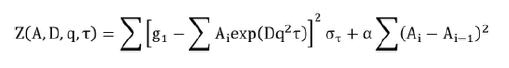Large alpha values (0.1) restrict the spikiness of the solution, resulting in smooth distributions. Small alpha values (0.0001) lessen the weighting or significance of the derivative term, consequently producing more spiky distributions. The alpha parameter therefore, can be defined as relatively representative of the anticipated level of noise in the measured correlogram.

An examples of the risks linked to the use of an overly aggressive alpha parameter can be seen in Figure 4. The findings displayed are those for 0.3 mg/ml lysozyme in PBS. In PBS, lysozyme is recognized as existing as a combination of oligomers – monomer, dimer, trimer, etc.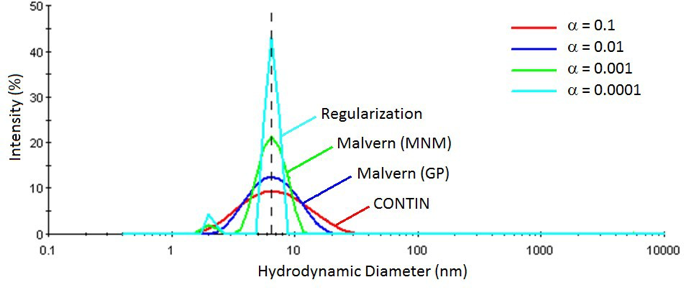Figure 4. Influence of the alpha parameter on DLS measured particle size distributions for 0.3 mg/ml lysozyme in PBS at pH 6.8.

As is clear from the findings displayed below, the measured average size of the protein distribution is not dependent on the alpha parameter chosen.

If the General Purpose algorithm is chosen, with an alpha value (α) of 0.01, the algorithm produces a precise intensity weighted mean size of the unresolved oligomeric distribution, with the peak width being illustrative of the anticipated DLS polydispersity for a mixture of protein oligomers.

The more aggressive algorithms, with α < 0.01, falsely squeeze the peak, dropping data relating to the unresolved components, as well as producing a phantom peak at circa 2 nm.

This phantom peak is a result of the signal to noise ratio innate to dilute protein measurements, in combination with an underestimate of that noise using an aggressive alpha parameter. Indeed, it was users of DLS studying dilute proteins who first used the term ‘ghost peaks’ to label phantom peaks resulting from the use of an overly aggressive NNLS algorithm.

There are four central enhancements offered by the MADLS and particle concentration data collection method that is built into the new Zetasizer Ultra system, when compared to traditional single angle DLS measurements.

Adaptive Correlation – this lessens levels of noise in the measured correlogram by measuring for shorter runs times and separating correlograms that are not statistically matching.

Combining back-scatter (BS), side-scatter (SS), and forwardscatter (FS) Correlograms –  this heightens the sensitivity to particles in scattering intensity minimum areas by coupling correlograms measured in backscatter, 90-degree scattering, and forward scattering angles.

High Resolution NNLS Algorithm – this increases the quantity of size bins used in the NNLS fitting and engages a multi-angle fitting technique to enable the extraction of additional particle size data from unresolved components within DLS peaks.

Laser Intensity Calibration – this removes the necessity to know or measure the incident laser intensity needed to calculate concentration by calibrating the system with a standard (toluene).

When employed together, these data collection and analysis enhancements result in more highly resolved and robust DLS particle size measurements by lessening the noise in the correlogram and ensuing inherent peak broadening, enabling the extraction of additional data from unresolved near in size components, and increasing confidence in ensuing concentration calculations.

## Multi-Angle Dynamic Light Scattering

The MADLS approach1, 2, 3 expands on the single-angle method, but with the addition of numerous measurement angles to the matrix transform. The autocorrelation functions are concatenated, as well as the scattering matrices, to yield the below system of linear equations, in which θ1 denotes the primary angle, θ2 denotes the secondary angle, etc.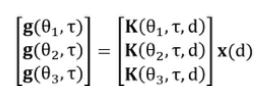Where data is being combined from a number of angles, it is paramount that the weighting inherent in the PSD (particle size distribution) is preserved. As such, the scattering matrix for each measurement angle is weighted by the anticipated scattered intensity.

In the Zetasizer Ultra, the PSD can be seen as a backscatter-equivalent intensity-weighted PSD or as a volume-weighted distribution, which is not dependent on the scattering angle. The scattered intensity (specifically differential scattering cross-section) is determined through the Mie theory and necessitates an understanding of the material and dispersant refractive index properties.

In the absence of the appropriate optical properties, the MADLS algorithm will fail to converge on the real solution.

In deriving the solution, the NNLS algorithm aims to lessen the magnitude of the residual (variance between the measured autocorrelation function and the prediction). Every angular contribution to the residual is weighted independently to account for the result uncertainty anticipated in that angle.

The techniques used in a MADLS and particle concentration measurements can be described as follows:

1. Use adaptive correlation to measure the correlograms for the sample at BS, SS, & F

2. Use the enhanced resolution MADLS NNLS algorithm to deconvolute the multi-angle system of linear equations to derive an intensity weighted particle size distribution;

a) Determine the anticipated BS, SS and FS correlograms using known particle refractive index values and the Mie theory

b) Determine the fit deviations for all correlograms

c) Using an iterative least squares process, adjust the particle size distribution and recalculate the anticipated correlograms and fit deviations, repeating until the total number of deviations is minimized

3. Convert the best fit intensity weighted particle size distribution into mass/ volume and number distributions, utilizing the known refractive index of the particles and the Mie theory

4. Employ the intensity distribution to calculate the portion of the total scattering intensity ascribed to each size component and determine the concentration of each component, by comparison of component scattering to the toluene scattering reference

The integration of adaptive correlation is the MADLS solution to low DLS resolution (stemming from noise in the correlogram). In adaptive correlation, the lengthier run times used in old-style DLS measurements are substituted for short runs.

Noise is kept to a minimum by separating correlograms that are not statistically equal, producing a high quality, low noise average correlogram which can be used to calculate the particle size distribution. This reduction in noise enables use of an enhanced resolution NNLS algorithm, with detected resolution limits being around 2 to 1 on the size, as opposed to the previously seen 3 to 1.

### Multi-Angle Correlograms

The application of multi-angle correlogram collection in the novel MADLS technique increases detection of low concentration weakly scattering particles in the scattering intensity minimum areas.

Particles which are troublesome to detect in the backscatter mode are easily detected at the other angles, particularly the forward angle, with no intensity minima until the particle size surpasses ~ 900 nm.

Additionally, multi angle correlogram collection results in a reduction in correlogram noise (or ambiguity) and ensuing inherent peak broadening, offering additional justification for the employment of the enhanced resolution MADLS NNLS algorithm.

### Enhanced Resolution NNLS Algorithm

The MADLS enhanced resolution algorithm employs a greater number of (smaller) size bins when compared to traditional single angle DLS NNLS algorithms.

This increased quantity of size bins is justified by the decrease in the correlogram noise which results from the integration of adaptive correlation and multi angle correlogram collection, delivering extraction of more highly resolved size data without inherent peak broadening or any false squeezing of the peak resulting from an overly aggressive alpha parameter.

As every correlogram is an independent measurement of the sample, angle-specific noise is not apparent in the result, since it is not common to all angles. The final result is a higher resolution intensity size distribution and a number transform that is more demonstrative of the genuine distribution of particles in the sample.

### Laser Intensity Calibration

Use of the overall intensity and DLS measured intensity size distribution to determine particle concentration is considered problematic due to correlogram noise and ambiguity in traditional single angle DLS measurements.

Correlogram noise compounds into inherent peak broadening, which subsequently compounds into false skewing of the number distribution toward imagined size components, leading to inaccurately calculated concentration values.

However, with the decrease in correlogram noise and higher resolution distributions resulting from the MADLS technique, calculation of the particle concentration from the DLS measured scattering intensity and the derived intensity weighted size distribution is far less troublesome. Nevertheless, knowledge of the incident laser intensity is required.

The concentration of particles in a solution can be determined from the scattering intensity using the expression displayed below, in which:

K1 is a known geometric constant, Io is the incident intensity, IA is the sample analyte scattering intensity (sample scattering – buffer scattering), d is the particle diameter, ño is the dispersant refractive index, ñP is the particle refractive index, λ is the incident wavelength, q is the observation or scattering angle related to the transmitted incident beam, C is the concentration in units of particles per unit volume, and the function f signifies the Mie theory.

For a stationary optical configuration and particles of known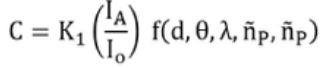optical properties, each parameter in the above expression is known, aside from the incident intensity (Io). As direct measurement of Io is challenging, it is usually defined using a scattering reference of known Rayleigh ratio (Rθ ), for example, toluene, using the expression shown here, where IT is the scattering intensity of toluene.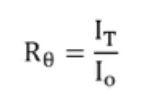After Io has been identified, determining the particle concentration in the sample is a simple process, with the intensity size distribution determining the percentage of the total scattering intensity ascribed to each size bin within the distribution.

The concentration of each particle family can then be calculated using the previous concentration expression in combination with the particle refractive index and Mie theory.

A mix of polystyrene spheres with diameters of 150 nm and 300 nm and comparable scattering intensities were prepared in a 10 mM aqueous solution of NaCl in order to demonstrate the resolution enhancements in the MADLS technique over old-style single angle DLS.

Figure 5 displays the single-angle intensity weighted particle size distributions results (backscatter, side scatter and forward scatter). Just one population is apparent and the size is variable.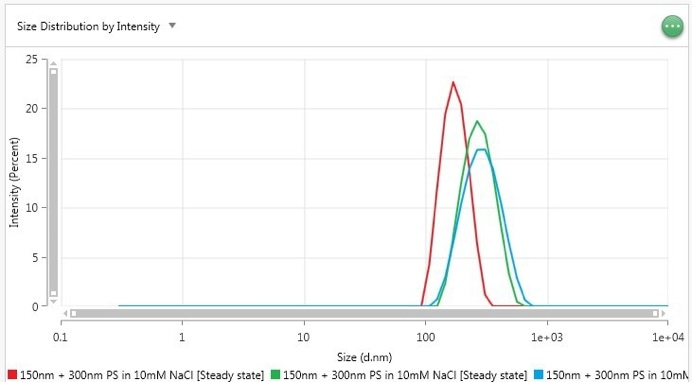Figure 5. Intensity-weighted particle size distribution of a 150 and 300 nm polystyrene sphere dispersion measured using DLS in backscatter (red), side scatter (green) and forward scatter (blue).

The background information offered earlier can help to understand these single angle DLS results. The 1st intensity minimum at a backscatter angle is around 300 nm. As a result of the lowered scattering intensity of the 300 nm component in backscatter, it is indistinguishable from random correlogram noise, with the 150 nm latex scattering dominating the intensity PSD results.

On the side and forward scattering angles, the 300 nm signal dominates both the correlograms and the derived particle size distributions. The variance in the widths or polydispersity of the side and forward scatter results can be ascribed to the known physical polydispersity of the 300 nm latex standard, which holds a small quantity of somewhat larger latex spheres.

The greater level of scattering seen with these larger particles at low angle leads to a spreading of the unresolved size peak and an ensuing increase in the peak mean.

Figure 6 displays the multi-angle MADLS result, and demonstrates a distinct peak corresponding to each component of the mixture, with near baseline resolution attained for a 2:1 ratio by size.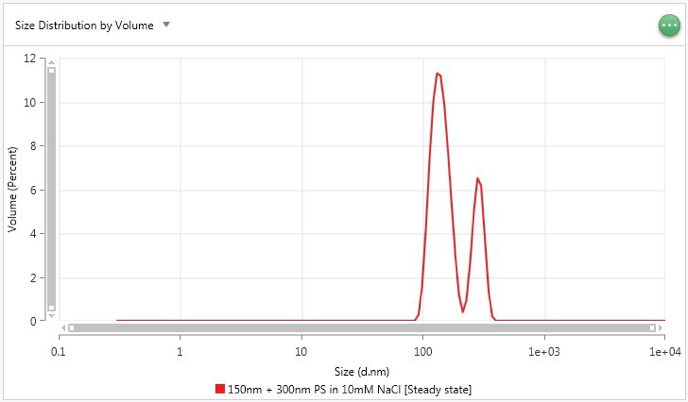Figure 6. Volume-weighted particle size distribution of a 150 and 300 nm polystyrene sphere dispersion measured using the Zetasizer Ultra MADLS technique.

Although the MADLS technique offers enhancements in DLS component resolution, limits on the quantity of information that can be extracted, as well as restrictions on sample suitability, remain.

Particle size and shape hold a strong bearing on the concentration calculations, thus the particles must be spherical and great precision in the intensity weighted particle size distribution is vital. It is essential that restricted diffusion effects (sample viscosity) be accounted for, as they will result in significant errors in the calculated concentrations.

It is not possible to correct electrostatic repulsion effects on the apparent particle size, therefore, samples demonstrating electrostatic repulsion (increased diffusion) are inappropriate for concentration measurements, with substantial inaccuracy anticipated within the mass and number distributions.

Although the MADLS technique keeps inherent broadening in the intensity size peaks to a minimum, the limiting assumptions relating to particle optical properties in the intensity to mass transform are still applicable, with an assumption that the particles are spherical with a homogeneous and known refractive index.

While the homogeneous particle assumption may be effective for core-shell type particles, such as liposome, viruses, coated nanoparticles, etc., it would still require determination of an average particle refractive index value suitable for the sample type and size.

As the concentration range must meet the requirements of each detection angle, the constraint of MADLS to measure the sample at three distinct angles limits the concentration range that is measurable.

To illustrate, turbid samples are inappropriate, since such samples require NIBS technology which is possible in backscatter only. Samples with large particles (i.e. larger than 500 nm diameter) will only be measurable following precise optimization of the concentration, if at all.

## Conclusion

The MADLS method has been described for users with an interest in how the measurement data is collated and studied. The central basis of the enhanced resolution over single-angle measurements is a reduction in correlogram noise, arising from three mechanisms:

1. Incorporation of adaptive correlation, which makes use of short correlogram run times and partitions correlograms that are not statistically equal from the steady state result.
2. Incorporation of multi angle correlogram measurements, which improves detection of low concentration or weakly scattering particles in the scattering intensity minimum areas and limits the solution, so that only common components from all autocorrelation functions are assimilated.
3. Improved resolution transformation over three distinct correlogram measurements

The decrease in correlogram noise enables use of an enhanced resolution NNLS algorithm, which makes use of a greater quantity of size bins (= small bin sizes) and fits to data from various measurement angles at the same time.

This fitting method circumvents or diminishes inherent peak broadening or any artificial squeezing of the peak as a result of an overly aggressive alpha parameter, leading to a higher resolution intensity size distribution that is more indicative of the true distribution of particles in the sample.

This MADLS technique enables users of the Zetasizer Ultra to collect further insight into their sample by offering a result which is independent of angles, bridging the gap between dynamic light scattering and high-resolution methods such as SEC or TEM.

1. P.G. Cummins, E.J. Staples, Particle Size Distributions Determined by a “Multiangle” Analysis of Photon Correlation Spectroscopy Data, Langmuir, 1987, 3, 1109-1113
2. G. Bryant, J.C. Thomas, Improved Particle Size Distribution Measurements Using Multiangle Dynamic Light Scattering, Langmuir, 1995, 11, 2480-2485
3. R. Xu, Particle Characterization: Light Scattering Methods, Springer, 257-258, Chapter 5, 2000This information has been sourced, reviewed and adapted from materials provided by Particulate Systems.

## Citations

• APA

Particulate Systems. (2020, January 09). Using MADLS for Improved Component Resolution. AZoM. Retrieved on June 02, 2020 from https://www.azom.com/article.aspx?ArticleID=17975.

• MLA

Particulate Systems. "Using MADLS for Improved Component Resolution". AZoM. 02 June 2020. <https://www.azom.com/article.aspx?ArticleID=17975>.

• Chicago

Particulate Systems. "Using MADLS for Improved Component Resolution". AZoM. https://www.azom.com/article.aspx?ArticleID=17975. (accessed June 02, 2020).

• Harvard

Particulate Systems. 2020. Using MADLS for Improved Component Resolution. AZoM, viewed 02 June 2020, https://www.azom.com/article.aspx?ArticleID=17975.

## Tell Us What You Think